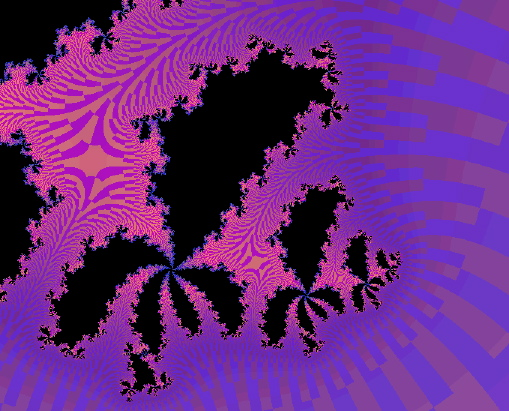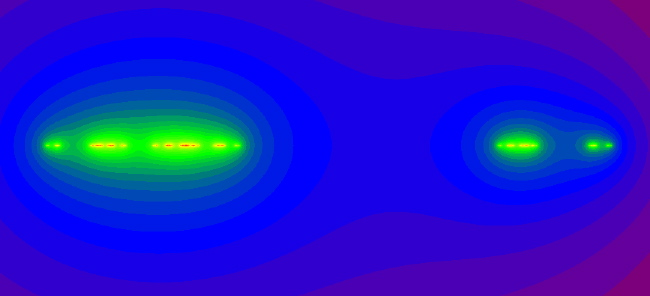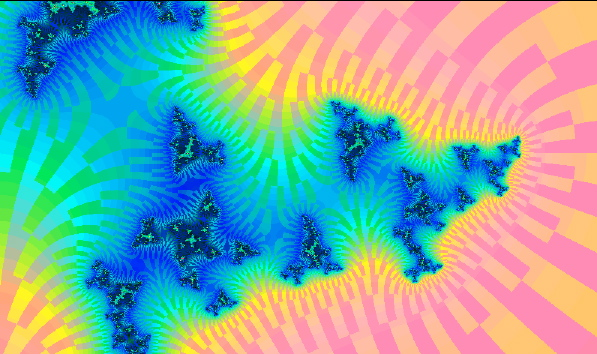Henon Map Dynamical Space Pics: 2

The following images were all created using FractalAsm, and the Henon_Unstable_Manifold definition.

These images show parameterized slices of the unstable manifold of a saddle fixed point of a Henon map, H. Points are drawn black if their forward orbit under H appears to be bounded, or else they are colored according to rate of escape. The saddle fixed point is the rightmost tip of the black.above: (c=0.15625+0.5423i, a=0.2375+0.90625).
You will need something like 1000 iterations to see the channels.

below: (c=0.362+0.3414i,a = 0.3). We smell period 5.
The checkerboard pattern illustrates exernal rays
(the "split" option in FractalAsm enables this coloration).below: (c=-3, a=-.34). This is a "real horseshoe".below: (c=0.1+0.7i, a=.3). Another interesting horseshoe, with external angles checkered.